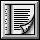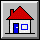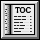5.8 Class INTEGER`indexing`
`description: "Integer values"`
`expanded class interface`
`INTEGER`
`feature -- Access`
```hash_code: INTEGER is
-- Hash code value
-- (From HASHABLE.)
ensure
good_hash_value: Result >= 0```
```one: like Current
-- Neutral element for "*" and "/"
-- (From NUMERIC.)
ensure
Result_exists: Result /= Void;
value: Result = 1```
```sign: INTEGER
-- Sign value (0, -1 or 1)
ensure
three_way: Result = three_way_comparison (zero)```
```zero: like Current
-- Neutral element for "+" and "-"
-- (From NUMERIC.)
ensure
Result_exists: Result /= Void;
value: Result = 0```
`feature -- Comparison`
```infix "<" (other: like Current): BOOLEAN
-- Is other greater than current integer?
-- (From COMPARABLE.)
require
other_exists: other /= Void
ensure
asymmetric: Result implies not (other < Current)```
```infix "<=" (other: like Current): BOOLEAN
-- Is current object less than or equal to other?
-- (From COMPARABLE.)
require
other_exists: other /= Void
ensure
definition: Result = (Current < other) or is_equal (other);```
```infix ">=" (other: like Current): BOOLEAN
-- Is current object greater than or equal to other?
-- (From COMPARABLE.)
require
other_exists: other /= Void
ensure
definition: Result = (other <= Current)```
```infix ">" (other: like Current): BOOLEAN
-- Is current object greater than other?
-- (From COMPARABLE.)
require
other_exists: other /= Void
ensure
definition: Result = (other < Current)```
```max (other: like Current): like Current
-- The greater of current object and other
-- (From COMPARABLE.)
require
other_exists: other /= Void
ensure
current_if_not_smaller: (Current >= other) implies (Result = Current)
other_if_smaller: (Current < other) implies (Result = other)```
```min (other: like Current): like Current
-- The smaller of current object and other
-- (From COMPARABLE.)
require
other_exists: other /= Void
ensure
current_if_not_greater: (Current <= other) implies (Result = Current)
other_if_greater: (Current > other) implies (Result = other)```
```three_way_comparison (other: like Current): INTEGER
-- If current object equal to other, 0; if smaller,
-- -1; if greater, 1.
-- (From COMPARABLE.)
require
other_exists: other /= Void
ensure
equal_zero: (Result = 0) = is_equal (other);
smaller: (Result = 1) = Current < other;
greater_positive: (Result = -1) = Current > other```
`feature -- Status report`
```divisible (other: like Current): BOOLEAN
-- May current object be divided by other?
-- (From NUMERIC.)
require
other_exists: other /= Void
ensure
value: Result = (other /= 0)```
```exponentiable (other: NUMERIC): BOOLEAN
-- May current object be elevated to the power other?
-- (From NUMERIC.)
require
other_exists: other /= Void
ensure
safe_values: (other.conforms_to (Current) or
(other.conforms_to (0.0) and (Current >= 0)))
implies Result```
`feature -- Basic operations`
```abs: like Current
-- Absolute value
ensure
non_negative: Result >= 0;
same_absolute_value: (Result = Current) or (Result = - Current)```
```infix "*" (other: like Current): like Current
-- Product by other
-- (From NUMERIC.)
require
other_exists: other /= Void```
```infix "+" (other: like Current): like Current
-- Sum with other
-- (From NUMERIC.)
require
other_exists: other /= Void
ensure
result_exists: Result /= Void;
commutative: equal (Result, other + Current)```
```infix "-" (other: like Current): like Current
-- Result of subtracting other
-- (From NUMERIC.)
require
other_exists: other /= Void
ensure
result_exists: Result /= Void```
```infix "/" (other: like Current): DOUBLE
-- Division by other
require
other_exists: other /= Void;
good_divisor: divisible (other)
ensure
result_exists: Result /= Void```
```infix "//" (other: like Current): like Current
-- Integer division of Current by other
-- (From "/" in NUMERIC.)
require
other_exists: other /= Void;
good_divisor: divisible (other)
ensure
result_exists: divisible (other)```
```infix "\\" (other: like Current): like Current
-- Remainder of the integer division of Current by other
require
other_exists: other /= Void;
good_divisor: divisible (other)
ensure
result_exists: Result /= Void```
```infix "^" (other: NUMERIC): DOUBLE
-- Integer power of Current by other
-- (From NUMERIC.)
require
other_exists: other /= Void;
good_exponent: exponentiable (other)
ensure
result_exists: Result /= Void```
```prefix "+": like Current
-- Unary plus
-- (From NUMERIC.)
ensure
result_exists: Result /= Void```
```prefix "-": like Current
-- Unary minus
-- (From NUMERIC.)
ensure
result_exists: Result /= Void```
`feature -- Output`
```out: STRING
-- Printable representation of current object
-- (From GENERAL.)```
`invariant`
```irreflexive_comparison: not (Current < Current);
neutral_addition: equal (Current + zero, Current);
self_subtraction: equal (Current - Current, zero);
neutral_multiplication: equal (Current * one, Current);
self_division: divisible (Current) implies equal (Current / Current, one)
sign_times_abs: equal (sign * abs, Current)```
`end`

 Copyright © 1995, Nonprofit International Consortium for Eiffel mailto:nice@atlanta.twr.com Last Updated: 26 October 1997